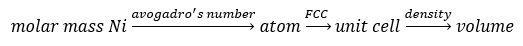# Problem: Nickel has a face-centered cubic unit cell. The density of nickel is 6.84 g/cm 3. Calculate a value for the atomic radius of nickel.

###### FREE Expert Solution

First step is to find the volume of the unit cell from the information given:Recall that a Face-Centered Cubic (FCC) unit cell has 4 atoms per unit cell.

Density = 6.84 g/cm3
Molar mass Ni = 58.69 g/mol

Calculate volume:

79% (334 ratings)###### Problem Details

Nickel has a face-centered cubic unit cell. The density of nickel is 6.84 g/cm 3. Calculate a value for the atomic radius of nickel.